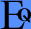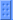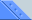More Skins Information EMail BackDon't Know No Math!?Want to learn more about these mathematical functions? Try on of these: Discovery's WebMath - Good and simple explanations. Dave's Math Tables - More professional. Math for Morons Like Us - Simple explanations AND high level. All together, Equalizer has 54 Functions, Operators and Constants.OperatorsOperators come between two expressions. Equalizer supports these operators: X + Y - add: sum of X and Y X - Y - subtract: difference of X from Y (6-4=2) X * Y - multiply: X times Y (2*3=6) X / Y - divide: X's in Y (14/4=3.5) X % Y - percents: X percent out of Y (15%20=3) X ^ Y - power: Xy (2^3=8) X div Y - whole Y's in X (10 div 3 = 3) X mod Y - X in module Y (10 mod 3 = 1) X t Y - base X square of Y (3 t 8=2) X e± Y - exponent: X*10y (3.24e+3 = 3.24*103 = 3240)ConstantsUse a constant in the same way you'd use any number: pi = π ≈ 3.1415926535897 phi = φ ≈ 1.6180339887498 e ≈ 2.71828182845905    Note: e+ and e- are used for exponents. x - in future versions, x will be used to draw a graph of the function.FunctionsFunctions come before or after expressions. Equalizer supports these functions: tX - Square Root (t9=3) [x] - Absolute Value ( [4-6] = 2 ) LogX - Logarithm (Base 10, Log10x) LnX - Natural Logarithm (Logex) FixX - The whole part of a number ( fix(3.9)= 3, fix(-2.8)= -2 ) IntX - The integer that is smaller than x, or equal to it ( int(1.9)= 1, int(-1.1)= -2 ) RoundX - Nearest integer ( round(1.2)= 1, round(-1.9)= -2)Functions: TrigonometrySinα- Sine of the angle       For All Trigonometry Functions: ASinα- Arc (invert) Sine SinHα- Hyperbolic Sine ASinHα- Arc Hyperbolic Sine Cosα - Cosine of the angle Tanα - Tangent of the angle Cotα - Cotangent of the angle Secα - Secant Cscα - Cosecant *Note: All Trigonometry functions are in Radians Radα - Convert a degree angle to radians Degα - Convert a radian angle to degreesFunctions: CombinatoricsX! - Factorial (4! = 1*2*3*4 = 24) C(n,m) - The number of ways to chose M items from a group of N. (C(4,2)=6) P(n,m) - The number of ways to chose and order M items from a group of N. (P(4,2)=12)Functions: StatisticsThese functions work on a series of numbers, and must be written with brackets.(use comma "," to seperate the items, or 'pipe' if comma is your decimal seperator, e.g. Max(3,5) or Sum(33|11) ) Max( X1,X2,X3...) - The maximum number of the series ( max(-5,2,3+1) = 4 ) Min( X1,X2,X3...) - The minimum number of the series ( min(-5,2,3+1) = -5 ) Sum( X1,X2,X3...) - The sum of all number ( sum(-5,2,3+1) = 1 ) Avg( X1,X2,X3...) - The average of all number ( avg(10,2,9) = 7 )